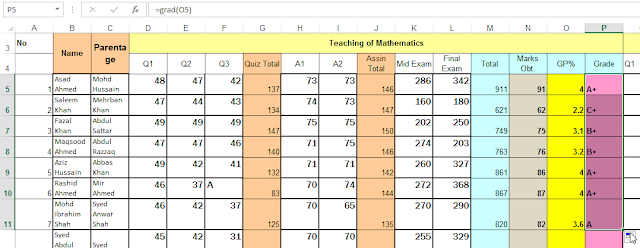# Grade calculation

## How to Calculate Grade using VBA Procedure in excel

We need to calculate Grade with college students examination numbers and We have an excel sheet.By opening the Microsoft VisualBasic Applications window you can access the VisualBasic (VBA) environment in Excel 2007-2013

To begin with, ensure that the VBA DEVELOPER tab is seen withinside the toolbar in Excel 2007. .

The DEVELOPER tab is the toolbar that has the buttons to open the VisualBasic (VBA) editor.
To display the Developer tab, click on on FILE withinside the menu and pick out Options beginning from the drop menu.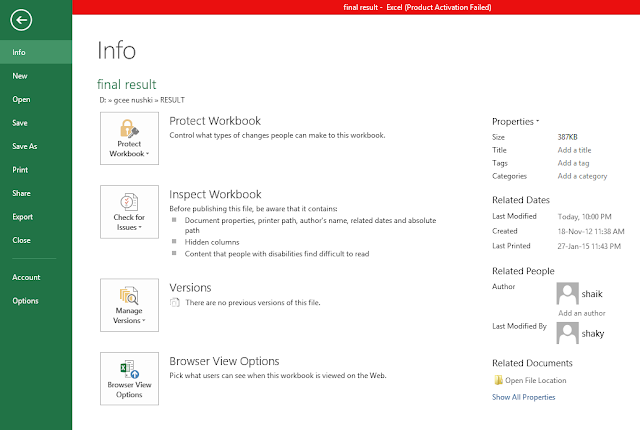At the factor whilst the Excel Options window indicates up, click on at the Customize Ribbon at the left.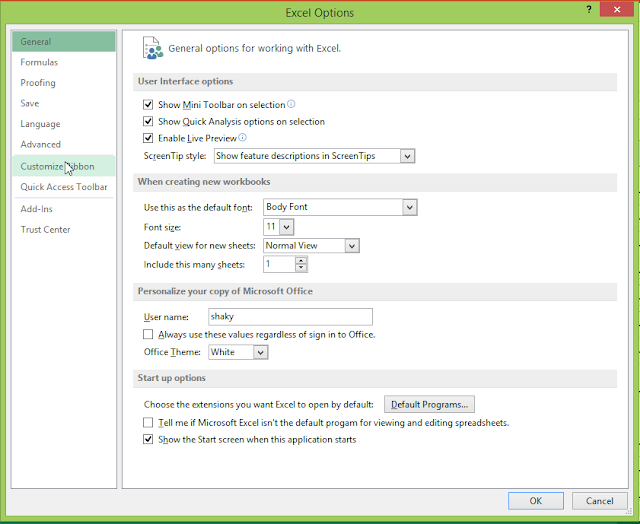Under the list of Customize Ribbon Main Tabs on the right, Select VBA Developer checkbox . Then click on the OK button.from the toolbar Select the VBA DEVELOPER tab at the top of the screen. Then in the Code group, click on the VisualBasic option.Presently the Microsoft VisualBasic VBA for Applications editor ought to seem and also you insert your VisualBasic VBA code.

From menu pick insert button then click on Module.The Applications editor of Visual Basic ought to to expose up then insert function Grade.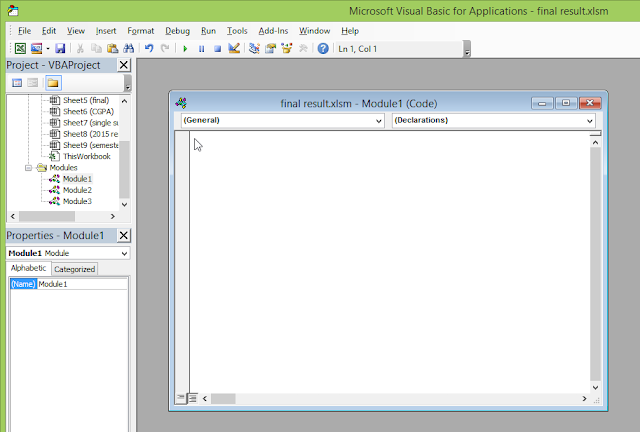```Function grad(alpha As Double)
If alpha = 4 Then
grad = "A+"
ElseIf alpha < 4 And alpha >= 3.4 Then
grad = "A"
ElseIf alpha < 3.4 And alpha >= 3.1 Then
grad = "B+"
ElseIf alpha < 3.1 And alpha >= 2.8 Then
grad = "B"
ElseIf alpha < 2.8 And alpha >= 2.4 Then
grad = "B-"
ElseIf alpha < 2.4 And alpha >= 2.1 Then
grad = "C+"
ElseIf alpha < 2.1 And alpha >= 1.8 Then
grad = "C"
ElseIf alpha < 1.8 And alpha >= 1.5 Then
grad = "C-"
ElseIf alpha < 1.5 And alpha >= 1 Then
grad = "D"
End If

End Function
```

After entereing the code then write procedure name called Grade then click save button and then close visualbasic editor.Now in cell O5 write formulation for N5 cell No to calculate GPA = gpa(N5),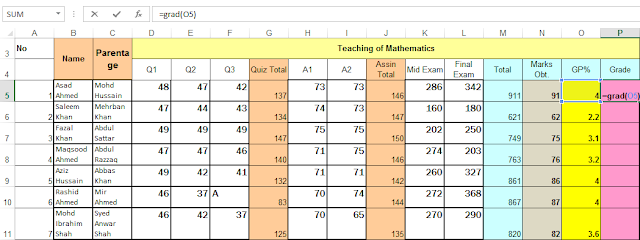Excel automated calculates the grade for given Student marks.copy this formula for other marks